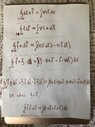# Alternate forms of Stokes' theorem? Are they correct? Are they named?

• I
Hiero
TL;DR Summary
I have a few 3D formulas akin to Stokes theorem taught to undergraduates (not the more powerful diff forms version).
The last formula is what I was going for, since it arises as the momentum flux in fluid dynamics, but in the process I came across the rest of these formulas which I’m not sure about.

The second equation is missing a minus sign (I meant to put [dA X grad(f)]).Are they correct? Do they have individual names? Or they’re all just roughly called ‘stokes theorems’?

Mentor
2022 Award
Stokes's theorem and all its variations are basically the fundamental theorem of calculus. It occurs in really many different forms, and many of them have names, e.g. Gauß's theorem or divergence theorem.

•vanhees71
Homework Helper
2022 Award
If these results are correct then they can be established by dotting one side with an arbitrary constant vector field. The aim in case of a volume integral is then to write the integrand as a divergence, and for a surface integral to write the integrand as a curl and apply the divergence theorem or Stokes' Theorem respectively. Now manipulate the result to obtain a dot product of the arbitrary constant field with an integral. You can then 'cancel' the dot product. Setting $d\mathbf{S} = \mathbf{n}\,dS$ where $\mathbf{n}$ is the outward unit normal and $d\mathbf{l} = \mathbf{t}\,d\ell$ where $\mathbf{t}$ is the unit tangent and taking advantage of the cyclic nature of the scalar triple product is also useful.

For example, to prove the first identity: Let $\mathbf{c}$ be an arbitrary constant vector. Then $$\nabla \cdot (\mathbf{f} \times \mathbf{c}) = \mathbf{c} \cdot (\nabla \times \mathbf{f}).$$ Now apply the divergence theorem: $$\mathbf{c} \cdot \int_V \nabla \times \mathbf{f}\,dV = \int_{\partial V} (\mathbf{f} \times \mathbf{c}) \cdot \mathbf{n}\,dS = \mathbf{c} \cdot \int_{\partial V} \mathbf{n} \times \mathbf{f}\,dS.$$ Hence $$\mathbf{c} \cdot \left(\int_V \nabla \times \mathbf{f}\,dV - \int_{\partial V} \mathbf{n} \times \mathbf{f}\,dS\right) = 0$$ and since this holds for all $\mathbf{c}$ it holds in particular for each of the cartesian standard basis vectors, and we must have $$\int_V \nabla \times \mathbf{f}\,dV = \int_{\partial V} \mathbf{n} \times \mathbf{f}\,dS.$$

•vanhees71